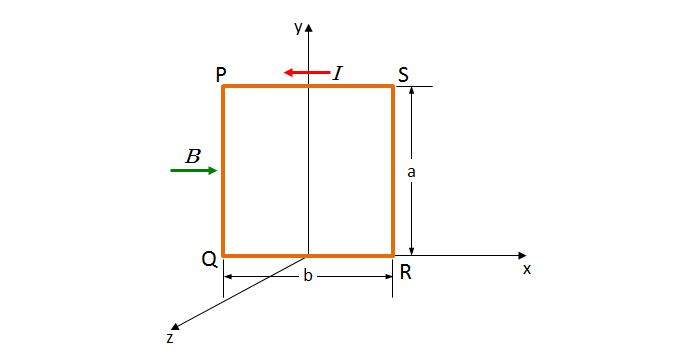# Torque from magnetic fieldA rectangular conducting wire $PQRS$ with current intensity $I$ is fixed on the $xy$-plane where a uniform magnetic field with magnetic intensity $B$ exists, as above. The direction of the magnetic field is $+x$ direction as indicated by the green arrow. What is the magnitude of the torque on the conducting wire by the magnetic field?

×